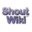Sayahna

## SFN:TeX4ht: TeX to HTML/MathML — conversion issues

The main problems that we faced when converting the TEX sources of Project Gutenberg Ebooks to HTML5/MathML formats are listed here.

## HTML

1. Text coded using {\itshape ...} not appeared as italic.
2. The html file where ToC entries should come is always blank. We have to copy the contents entries from title page to this page.

## MathML

1. The footnote counter in display equations appear in a new line without a link to its corresponding footnote text.
2. \textstyle does not work for fractions.
3. \intertext in {gather} is not rendered. It has converted in MathML, but rendering failed due to wrong tagging.
4. \limits in inline equations does not produce the desired output.
5. The equation number coded in \tag{} in starred display math environments (eg: equation*, eqnarray*, align* etc.) render without right alignment like normal equation number.
6. \makebox[][]{} does not produce any code.
7. The font of equation number differs for equations coded in {equation}, {eqnarray} or any amsmath environements like {align}, {gather} etc.
8. The alignment of equations coded in {aligned} environment completely lost when \textit was used instead of \mathit.
9. The above/belowskip of an equation is too small when it appears inside a list.

$ \newcommand{\Re}{\mathrm{Re}\,} \newcommand{\pFq}{{}_{#1}\mathrm{F}_{#2} \left( \genfrac{}{}{0pt}{}{#3}{#4} \bigg| {#5} \right)} $

We consider, for various values of $s$, the $n$-dimensional integral \begin{align}

 \label{def:Wns}
W_n (s)
&:=
\int_{[0, 1]^n}
\left| \sum_{k = 1}^n \mathrm{e}^{2 \pi \mathrm{i} \, x_k} \right|^s \mathrm{d}\boldsymbol{x}


\end{align} which occurs in the theory of uniform random walk integrals in the plane, where at each step a unit-step is taken in a random direction. As such, the integral \eqref{def:Wns} expresses the $s$-th moment of the distance to the origin after $n$ steps.

By experimentation and some sketchy arguments we quickly conjectured and strongly believed that, for $k$ a nonnegative integer \begin{align}

 \label{eq:W3k}
W_3(k) &= \Re \, \pFq32{\frac12, -\frac k2, -\frac k2}{1, 1}{4}.


\end{align} Appropriately defined, \eqref{eq:W3k} also holds for negative odd integers. The reason for \eqref{eq:W3k} was long a mystery, but it will be explained at the end of the paper.